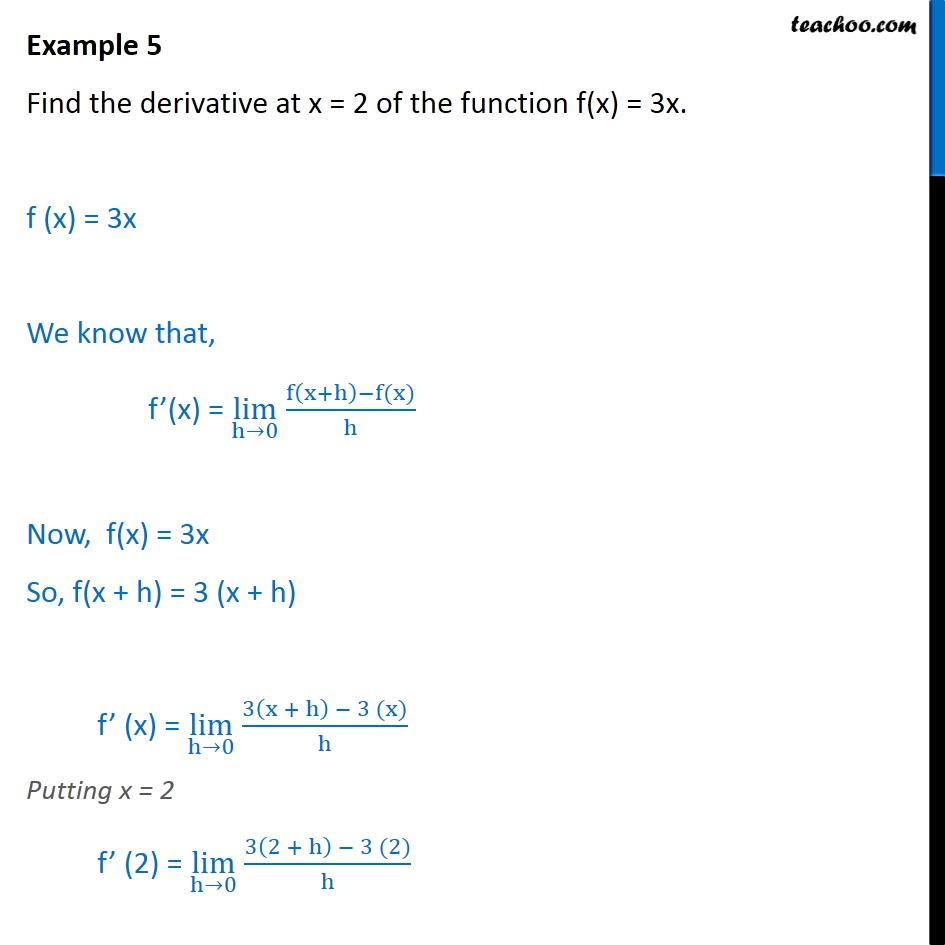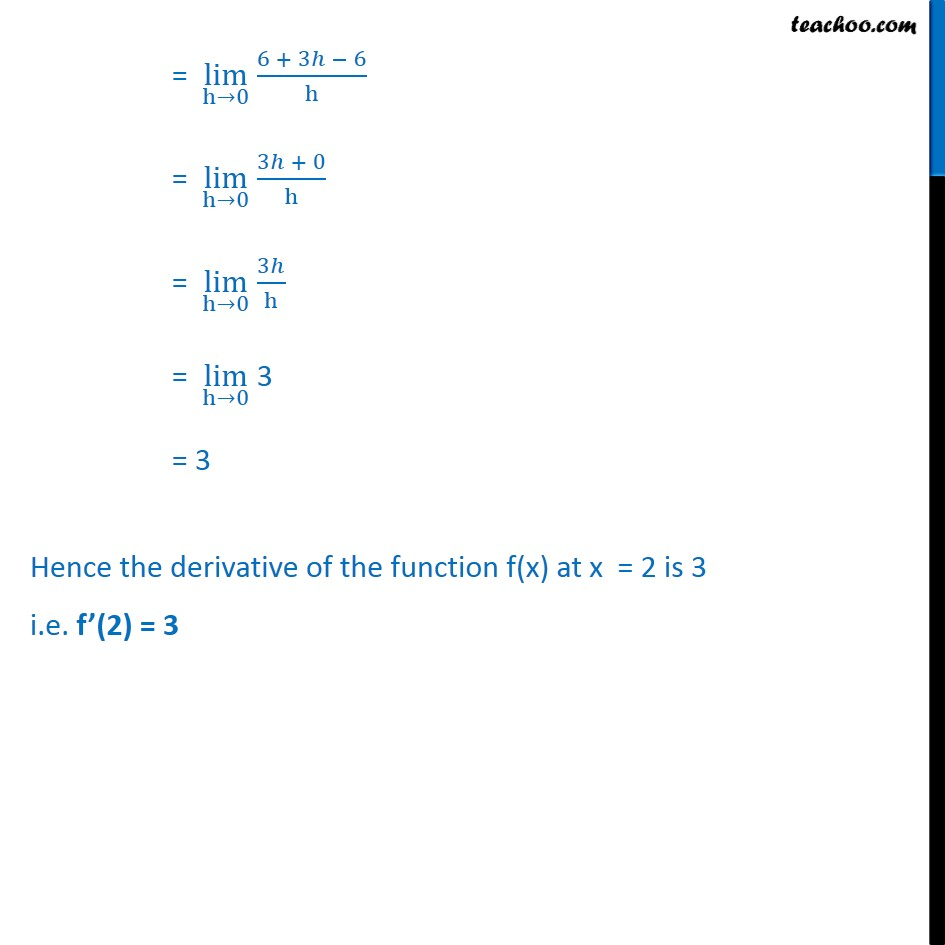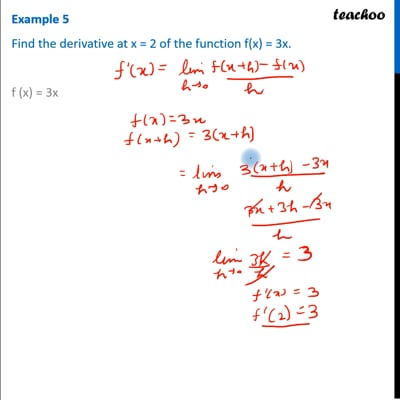Examples

Chapter 13 Class 11 Limits and Derivatives
Serial order wiseThis video is only available for Teachoo black users

Get live Maths 1-on-1 Classs - Class 6 to 12

### Transcript

Example 5 Find the derivative at x = 2 of the function f(x) = 3x. f (x) = 3x We know that, f’(x) = lim﷮h→0﷯ f﷮ x+h﷯−f(x)﷯﷮h﷯ Now, f(x) = 3x So, f(x + h) = 3 (x + h) f’ (x) = lim﷮h→0﷯ 3﷮ x + h﷯ − 3 (x)﷯﷮h﷯ Putting x = 2 f’ (2) = lim﷮h→0﷯ 3﷮ 2 + h﷯ − 3 (2)﷯﷮h﷯ = lim﷮h→0﷯ 6 + 3ℎ − 6﷮h﷯ = lim﷮h→0﷯ 3ℎ + 0﷮h﷯ = lim﷮h→0﷯ 3ℎ﷮h﷯ = lim﷮h→0﷯ 3 = 3 Hence the derivative of the function f(x) at x = 2 is 3 i.e. f’(2) = 3